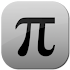# Full Scientific Calculator v1.84d

Contains Ads

Downloads: 144

Free

### Full Scientific Calculator / Screenshots### Full Scientific Calculator / Description

This calculator is also available in a faster ad-free version:
https://play.google.com/store/apps/details?id=com.jjsoftware.fullscientificcalculatorpro

This supercalculator contains within itself the complete functionality of today's high-end calculators. No feature was left behind during development. Featuring an automatically re-sizing navigable screen with unlimited digit support.

Its unique design allows you to comfortably write complicated equations while also enabling you to compose short and simple calculations fast. You can have a result bank of up to 1000 calculations of which you can always go back and edit.

Unparalleled in features its one-screen functionality makes it one of the simplest yet powerful calculators you will ever have the pleasure of operating.

Expanded feature list includes:

• Less than 3MB in size!
• Streamlined & fast ad-free experience
• Fully-formatted math that includes everything from fractions to equations
• Quadratic cubic and quartic polynomial equations with complex numbers
• 2nd 3rd and 4th degree linear equations
• Normal and hyperbolic trigonometry complete with inversion
• Normal and inverse cotangent secant & co-secant functions
• Unlimited number of digits
• Regular improper and mixed fractions
• Sexagesimal/DMS (degrees minutes seconds) calculations & conversions
• Decimal to/from fraction conversion
• Extremely high precision
• Result history up to 1000 answers
• Integration and differentiation calculus equations
• Matrices & vectors up to 3x3 cells with transposition inversion power scalar diagonal and arithmetic with complex numbers
• Algebraic equations
• Gamma function
• 34 Metric/Imperial conversions
• 44 scientific constants
• Boolean algebra including NOT OR AND and XOR
• Conversions and calculations between base 2 to 16
• Custom natural and base-10 logarithms
• Easy 4-directional navigation
• Answer display in decimal proper & improper fractions.
• Descriptive statistics functions including: mean median range midrange variance gini coefficient sum of squares trimean skewness kurtosis mean deviation standard deviation relative standard deviation/variance coefficient standard mean error root mean square (RMS) interquartile mean interquartile range. All answers are in "sample" format not "population".
• Multi-threaded calculations
• Scientific and engineering notation
• Factorial decomposition
• Permutations & Combinations
• Least common modifier and greatest common factor functions
• Modulus/remainder function
• Multiple angle types including degrees radians and gradians
• Factorials both integer and fractional
• Ordinary cubic and custom roots
• Random integers & decimals
• Plus/minus sign
• Absolution
• Summation function
• Product summation function
• Answer memorization
• Natural constants
• Frac & Int functions
• Percents

Want to know more? You can consult the brief user manual for detailed explanations of every feaure.

Bug reports suggestions or feature requests will be received at [email protected]

Show More >

### Full Scientific Calculator / What's New in v1.84d

Fixed a bug causing some OS 9 users to crash.

### Full Scientific Calculator / Tags

Recommended for You

Loading...